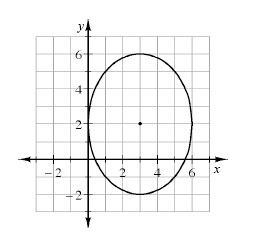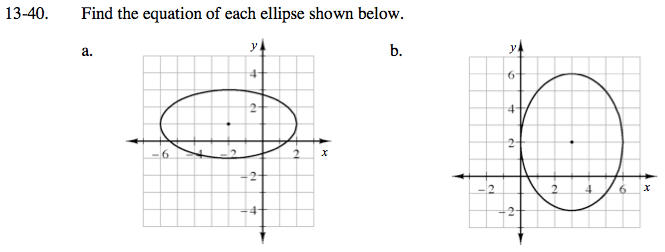### Home > A2C > Chapter 13 > Lesson 13.1.2 > Problem13-40

13-40.
1. Find the equation of each ellipse shown below. Homework Help ✎

1.2.Center at (− 2, 1)
Width: 8
Height: 4

$\frac{(x+2)^2}{16}+\frac{(y-1)^2}{4}=1$

See part (a).